# Creating plots in R using ggplot2 - part 9: function plots

written in

This is the ninth tutorial in a series on using ggplot2 I am creating with Mauricio Vargas Sepúlveda. In this tutorial we will demonstrate some of the many options the ggplot2 package has for plotting and customising functions.

If you enjoyed this blog post and found it useful, please consider buying our book! It contains chapters detailing how to build and customise all 11 chart types published on the blog, as well as LOWESS charts. The book is also actively maintained (unlike the series on the blog) and contains up-to-date ggplot and tidyverse code, and every purchase really helps us out with keeping up with new content.In this tutorial, we will work towards creating the function plot below. We will take you from a basic function plot and explain all the customisations we add to the code step-by-step.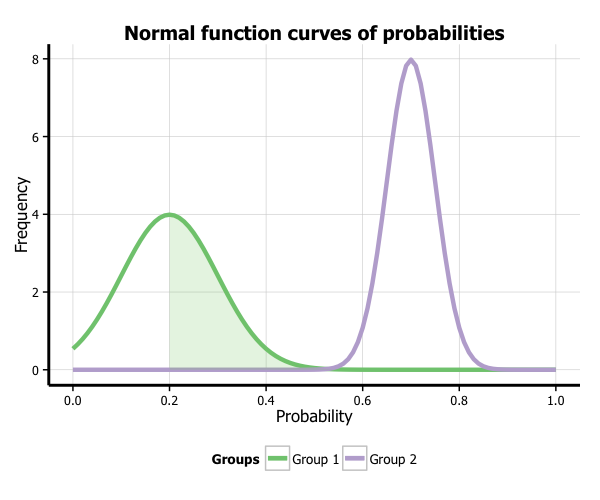### Basic normal curve

In order to create a normal curve, we create a ggplot base layer that has an x-axis range from -4 to 4 (or whatever range you want!), and assign the x-value aesthetic to this range (aes(x = x)). We then add the stat_function option and add dnorm to the function argument to make it a normal curve.

p9 <- ggplot(data.frame(x = c(-4, 4)), aes(x = x)) +
stat_function(fun = dnorm)
p9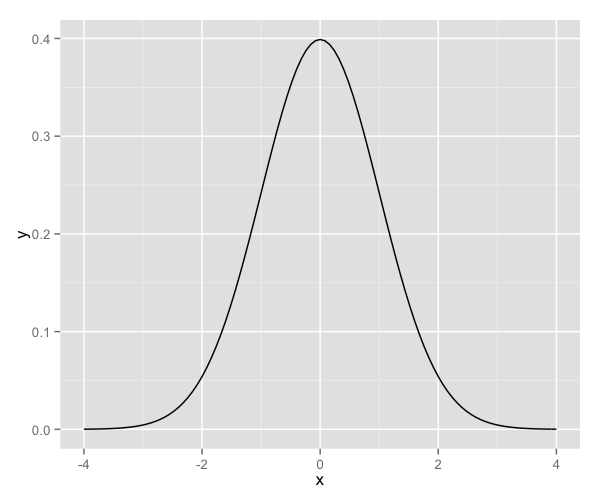### Basic t- curve

stat_function can draw a range of continuous probability density functions, including t (dt), F (df) and Chi-square (dchisq) PDFs. Here we will plot a t-distribution. As the shape of the t-distribution changes depending on the sample size (indicated by the degrees of freedom, or df), we need to specify our df value as part of defining our curve.

p9 <- ggplot(data.frame(x = c(-4, 4)), aes(x = x)) +
stat_function(fun = dt, args = list(df = 8))
p9You can also draw your own function, as long as it takes the form of a formula that converts an x-value into a y-value. Here we have plotted a curve that returns y-values that are the cube of x times a half:

cubeFun <- function(x) {
x^3 * 0.5
}

p9 <- ggplot(data.frame(x = c(-4, 4)), aes(x = x)) +
stat_function(fun = cubeFun)
p9### Plotting multiple functions on the same graph

You can plot multiple functions on the same graph by simply adding another stat_function() for each curve. Here we have plotted two normal curves on the same graph, one with a mean of 0.2 and a standard deviation of 0.1, and one with a mean of 0.7 and a standard deviation of 0.05. (Note that the dnorm function has a default mean of 0 and a default standard deviation of 1, which is why we didn’t need to explicitly define them in the first normal curve we plotted above.) You can also see we’ve changed the range of the x-axis to between 0 and 1.

p9 <- ggplot(data.frame(x = c(0, 1)), aes(x = x)) +
stat_function(fun = dnorm, args = list(0.2, 0.1)) +
stat_function(fun = dnorm, args = list(0.7, 0.05))
p9### Customising axis labels

Let’s move forward with this two function graph, and start tweaking the appearance. In order to change the axis labels, we have a couple of options. In this case, we have used the scale_x_continuous and scale_y_continuous options, as these have further customisation options for the axes we will use below. In each, we add the desired name to the name argument as a string.

p9 <- p9 + scale_x_continuous(name = "Probability") +
scale_y_continuous(name = "Frequency")
p9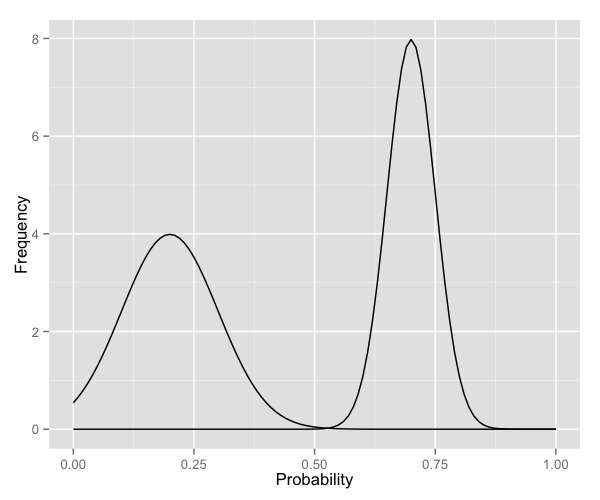### Changing axis ticks

The next thing we will change is the axis ticks. Let’s make the x-axis ticks appear at every 0.2 units rather than 0.25 using the breaks = seq(0, 1, 0.2) argument in scale_x_continuous. (The seq function is a base R function that indicates the start and endpoints and the units to increment by respectively. See help(seq) for more information.) We ensure that the x-axis begins and ends where we want by also adding the argument limits = c(0, 1) to scale_x_continuous.

p9 <- p9 + scale_x_continuous(name = "Probability",
breaks = seq(0, 1, 0.2),
limits=c(0, 1)) +
scale_y_continuous(name = "Frequency")
p9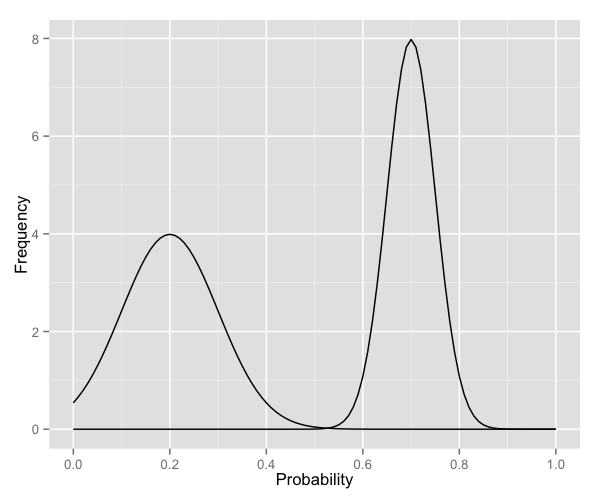To add a title, we include the option ggtitle and include the name of the graph as a string argument.

p9 <- p9 + ggtitle("Normal function curves of probabilities")
p9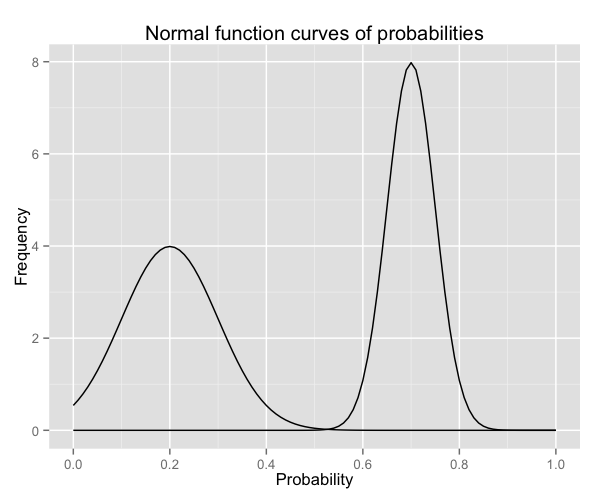### Changing the colour of the curves

To change the line colours of the curves, we add a valid colour to the colour arguments in stat_function. A list of valid colours is here.

p9 <- ggplot(data.frame(x = c(0, 1)), aes(x = x)) +
stat_function(fun = dnorm, args = list(0.2, 0.1),
colour = "deeppink") +
stat_function(fun = dnorm, args = list(0.7, 0.05),
colour = "dodgerblue3") +
scale_x_continuous(name = "Probability",
breaks = seq(0, 1, 0.2),
limits=c(0, 1)) +
scale_y_continuous(name = "Frequency") +
ggtitle("Normal function curves of probabilities")
p9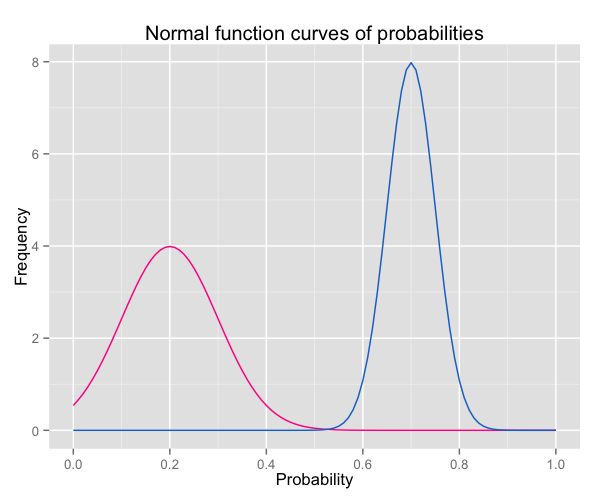If you want to go beyond the options in the list above, you can also specify exact HEX colours by including them as a string preceded by a hash, e.g., “#FFFFFF”. Below, we have called two shades of blue for the lines using their HEX codes.

p9 <- ggplot(data.frame(x = c(0, 1)), aes(x = x)) +
stat_function(fun = dnorm, args = list(0.2, 0.1),
colour = "#4271AE") +
stat_function(fun = dnorm, args = list(0.7, 0.05),
colour = "#1F3552") +
scale_x_continuous(name = "Probability",
breaks = seq(0, 1, 0.2),
limits=c(0, 1)) +
scale_y_continuous(name = "Frequency") +
ggtitle("Normal function curves of probabilities")
p9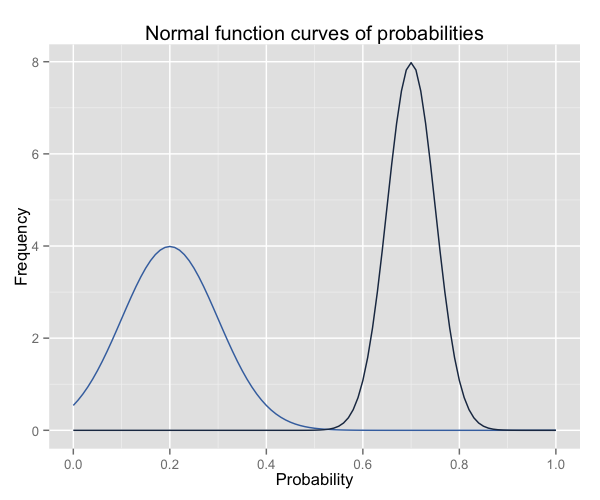As we have added two separate commands to plot the two function curves, ggplot does not automatically recognise that it needs to create a legend. We can make a legend by swapping out the colour argument in each of the stat_function commands for aes(colour = ), and assigning it the name of the group. We also need to add the scale_colour_manual command to make the legend appear, and also assign colours and a title.

p9 <- ggplot(data.frame(x = c(0, 1)), aes(x = x)) +
stat_function(fun = dnorm, args = list(0.2, 0.1),
aes(colour = "Group 1")) +
stat_function(fun = dnorm, args = list(0.7, 0.05),
aes(colour = "Group 2")) +
scale_x_continuous(name = "Probability",
breaks = seq(0, 1, 0.2),
limits=c(0, 1)) +
scale_y_continuous(name = "Frequency") +
ggtitle("Normal function curves of probabilities") +
scale_colour_manual("Groups", values = c("deeppink", "dodgerblue3"))
p9If you want to use one of the automatic brewer palettes, you can swap scale_colour_manual for scale_colour_brewer, and call your favouite brewer colour scheme. You can see all of the brewer palettes using display.brewer.all(5) As this command doesn’t allow you to assign a title to the legend, you can assign a title using labs(colour = "Groups").

p9 <- ggplot(data.frame(x = c(0, 1)), aes(x = x)) +
stat_function(fun = dnorm, args = list(0.2, 0.1),
aes(colour = "Group 1")) +
stat_function(fun = dnorm, args = list(0.7, 0.05),
aes(colour = "Group 2")) +
scale_x_continuous(name = "Probability",
breaks = seq(0, 1, 0.2),
limits=c(0, 1)) +
scale_y_continuous(name = "Frequency") +
ggtitle("Normal function curves of probabilities") +
scale_colour_brewer(palette="Accent") +
labs(colour = "Groups")
p9### Changing the size of the lines

As you can see, the lines are a little difficult to see. You can make them thicker (or thinner) using the argument size argument within stat_function. Here we have changed the thickness of each line to size 2.

p9 <- ggplot(data.frame(x = c(0, 1)), aes(x = x)) +
stat_function(fun = dnorm, args = list(0.2, 0.1),
aes(colour = "Group 1"), size = 1.5) +
stat_function(fun = dnorm, args = list(0.7, 0.05),
aes(colour = "Group 2"), size = 1.5) +
scale_x_continuous(name = "Probability",
breaks = seq(0, 1, 0.2),
limits=c(0, 1)) +
scale_y_continuous(name = "Frequency") +
ggtitle("Normal function curves of probabilities") +
scale_colour_brewer(palette="Accent") +
labs(colour = "Groups")
p9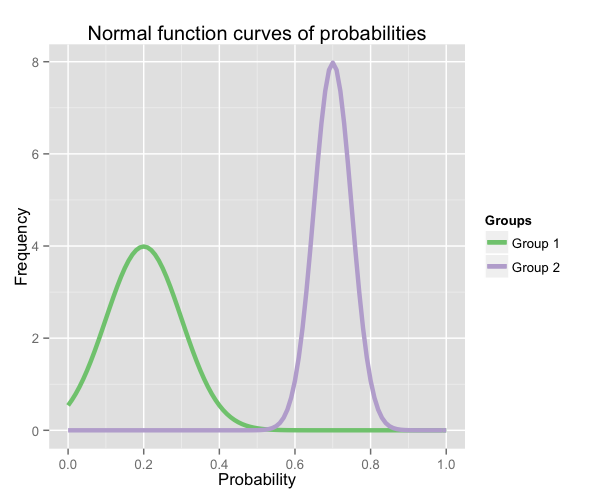### Using the white theme

As explained in the previous posts, we can also change the overall look of the plot using themes. We’ll start using a simple theme customisation by adding theme_bw() after ggplot(). As you can see, we can further tweak the graph using the theme option, which we’ve used so far to change the legend.

p9 <- p9 + theme_bw()
p9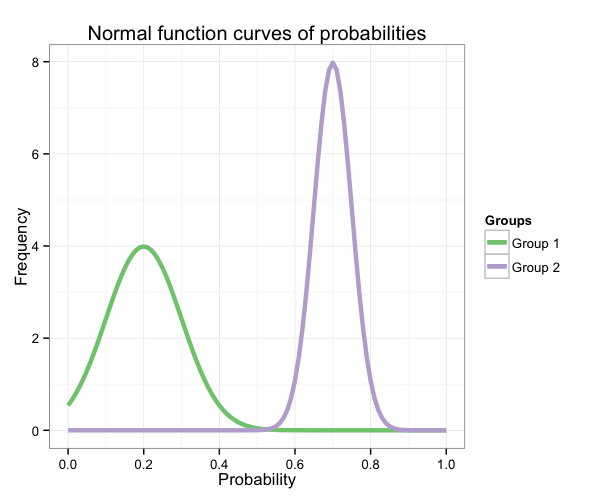### Creating an XKCD style chart

Of course, you may want to create your own themes as well. ggplot2 allows for a very high degree of customisation, including allowing you to use imported fonts. Below is an example of a theme Mauricio was able to create which mimics the visual style of XKCD. In order to create this chart, you first need to import the XKCD font, install it on your machine and load it into R using the extrafont package. These instructions are taken from here:

library(extrafont)

dest="xkcd.ttf", mode="wb")
system("mkdir ~/.fonts")
system("cp xkcd.ttf  ~/.fonts")
font_import(paths = "~/.fonts", pattern="[X/x]kcd")
fonts()


You can then create your graph:

p9 <- ggplot(data.frame(x = c(0, 1)), aes(x = x)) +
stat_function(fun = dnorm, args = list(0.2, 0.1),
aes(colour = "Group 1"), size = 1.5) +
stat_function(fun = dnorm, args = list(0.7, 0.05),
aes(colour = "Group 2"), size = 1.5) +
scale_x_continuous(name = "Probability",
breaks = seq(0, 1, 0.2),
limits=c(0, 1)) +
scale_y_continuous(name = "Frequency") +
ggtitle("Normal function curves of probabilities") +
scale_colour_brewer(palette="Set1") +
labs(colour = "Groups") +
theme(axis.line = element_line(size=1, colour = "black"),
panel.grid.major = element_blank(),
panel.grid.minor = element_blank(),
panel.border = element_blank(),
panel.background = element_blank(),
plot.title=element_text(size = 20, family="xkcd-Regular"),
text=element_text(size = 16, family="xkcd-Regular"),
axis.text.x=element_text(colour="black", size = 12),
axis.text.y=element_text(colour="black", size = 12))
p9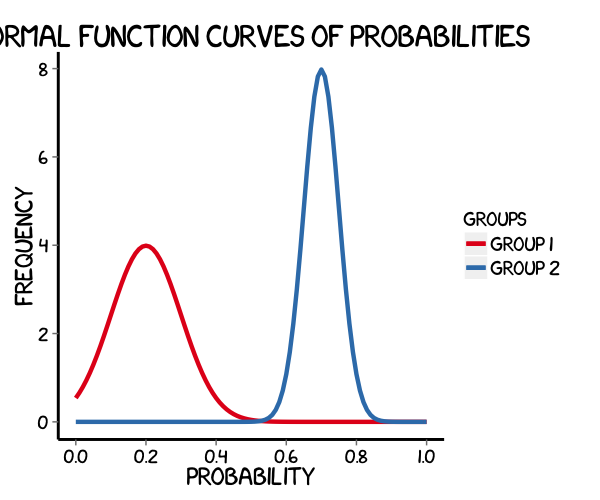### Using ‘The Economist’ theme

There are a wider range of pre-built themes available as part of the ggthemes package (more information on these here). Below we’ve applied theme_economist(), which approximates graphs in the Economist magazine.

library(ggthemes)

p9 <- ggplot(data.frame(x = c(0, 1)), aes(x = x)) +
stat_function(fun = dnorm, args = list(0.2, 0.1),
aes(colour = "Group 1"), size = 1.5) +
stat_function(fun = dnorm, args = list(0.7, 0.05),
aes(colour = "Group 2"), size = 1.5) +
scale_x_continuous(name = "Probability",
breaks = seq(0, 1, 0.2),
limits=c(0, 1)) +
scale_y_continuous(name = "Frequency") +
ggtitle("Normal function curves of probabilities") +
scale_colour_manual("Groups", values = c("#4271AE", "#1F3552")) +
theme_economist() +
theme(legend.position = "bottom", legend.direction = "horizontal",
legend.box = "horizontal",
legend.key.size = unit(1, "cm"),
plot.title = element_text(family="Tahoma"),
text = element_text(family = "Tahoma"),
axis.title = element_text(size = 12),
legend.text = element_text(size = 9),
legend.title=element_text(face = "bold", size = 9))
p9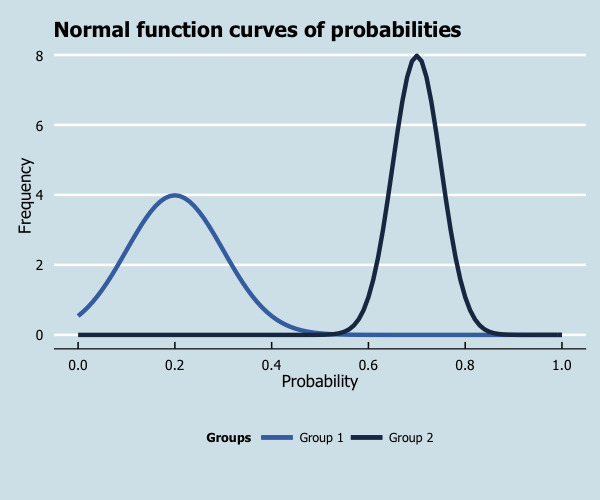As before, you can modify your plots a lot as ggplot2 allows many customisations. Here is a custom plot where we have modified the axes, background and font.

library(grid)

p9 <- ggplot(data.frame(x = c(0, 1)), aes(x = x)) +
stat_function(fun = dnorm, args = list(0.2, 0.1),
aes(colour = "Group 1"), size = 1.5) +
stat_function(fun = dnorm, args = list(0.7, 0.05),
aes(colour = "Group 2"), size = 1.5) +
scale_x_continuous(name = "Probability",
breaks = seq(0, 1, 0.2),
limits=c(0, 1)) +
scale_y_continuous(name = "Frequency") +
ggtitle("Normal function curves of probabilities") +
scale_colour_brewer(palette="Accent") +
labs(colour = "Groups") +
theme_bw() +
theme(axis.line = element_line(size=1, colour = "black"),
panel.grid.major = element_line(colour = "#d3d3d3"),
panel.grid.minor = element_blank(),
panel.border = element_blank(), panel.background = element_blank(),
plot.title = element_text(size = 14, family = "Tahoma", face = "bold"),
text=element_text(family="Tahoma"),
axis.text.x=element_text(colour="black", size = 9),
axis.text.y=element_text(colour="black", size = 9))
p9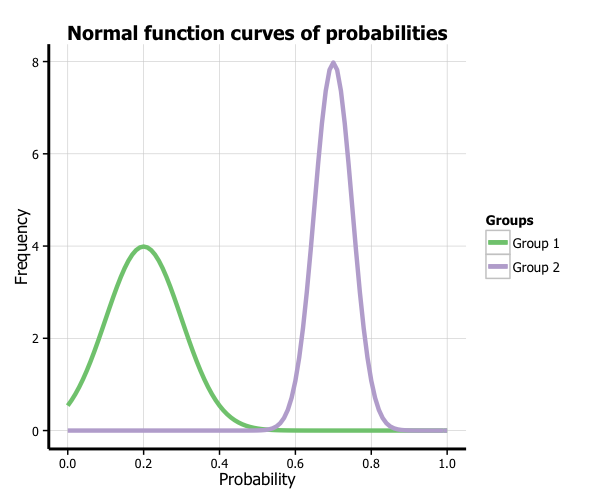### Adding areas under the curve

If we want to shade an area under the curve, we can do so by creating a function that generates a range of normal values with a given mean and standard deviation, and then only retains those values that lie within the desired range (by assigning NAs to everything outside of the range). In this case, we have created a shaded area under the group 1 curve which covers between the mean and 4 standard deviations above the mean (as given by 0.2 + 4 * 0.1). We then add another stat_function command to the graph which plots the area specified by this function, indicates it should be an area plot, and makes it semi-transparent using the alpha argument.

funcShaded <- function(x) {
y <- dnorm(x, mean = 0.2, sd = 0.1)
y[x < 0.2 | x > (0.2 + 4 * 0.1)] <- NA
return(y)
}

p9 <- p9 + stat_function(fun=funcShaded, geom="area", fill="#84CA72", alpha=0.2)
p9### Formatting the legend

Finally, we can format the legend by changing the position. We simply add the legend.position = "bottom" argument to the theme option, which moves the legend under the plot.

p9 <- ggplot(data.frame(x = c(0, 1)), aes(x = x)) +
stat_function(fun = dnorm, args = list(0.2, 0.1),
aes(colour = "Group 1"), size = 1.5) +
stat_function(fun = dnorm, args = list(0.7, 0.05),
aes(colour = "Group 2"), size = 1.5) +
scale_x_continuous(name = "Probability",
breaks = seq(0, 1, 0.2),
limits=c(0, 1)) +
scale_y_continuous(name = "Frequency") +
ggtitle("Normal function curves of probabilities") +
scale_colour_brewer(palette="Accent") +
labs(colour = "Groups") +
theme_bw() +
theme(axis.line = element_line(size=1, colour = "black"),
panel.grid.major = element_line(colour = "#d3d3d3"),
panel.grid.minor = element_blank(),
panel.border = element_blank(), panel.background = element_blank(),
plot.title = element_text(size = 14, family = "Tahoma", face = "bold"),
text=element_text(family="Tahoma"),
axis.text.x=element_text(colour="black", size = 9),
axis.text.y=element_text(colour="black", size = 9),
legend.position = "bottom")
p9